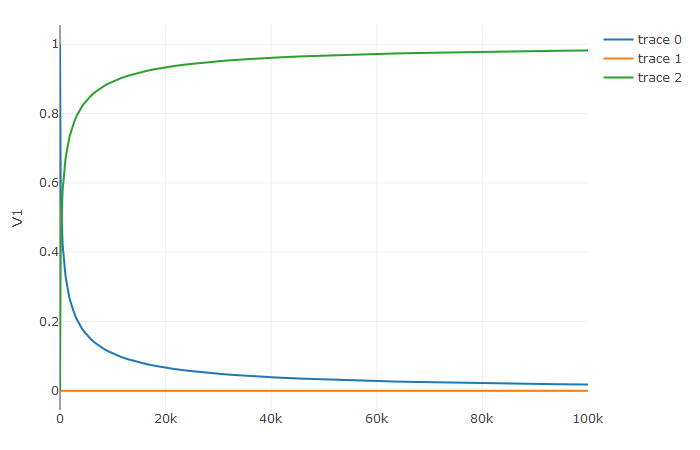A differential-algebraic equation is defined by an implicit function `f(du,u,p,t)=0`. All of the controls are the same as the other examples, except here you define a function which returns the residuals for each part of the equation to define the DAE. The initial value `u0` and the initial derivative `du0` are required, though they do not necessarily have to satisfy `f` (known as inconsistent initial conditions). The methods will automatically find consistent initial conditions. In order for this to occur, `differential_vars` must be set. This vector states which of the variables are differential (have a derivative term), with `false` meaning that the variable is purely algebraic.

This example shows how to solve the Robertson equation:

``````f <- function (du,u,p,t) {
resid1 = - 0.04*u              + 1e4*u*u - du
resid2 = + 0.04*u - 3e7*u^2 - 1e4*u*u - du
resid3 = u + u + u - 1.0
c(resid1,resid2,resid3)
}
u0 <- c(1.0, 0, 0)
du0 <- c(-0.04, 0.04, 0.0)
tspan <- c(0.0,100000.0)
differential_vars <- c(TRUE,TRUE,FALSE)
prob <- de\$DAEProblem(f,du0,u0,tspan,differential_vars=differential_vars)
sol <- de\$solve(prob)
udf <- as.data.frame(t(sapply(sol\$u,identity)))
plotly::plot_ly(udf, x = sol\$t, y = ~V1, type = 'scatter', mode = 'lines') %>%
``````Additionally, an in-place JIT compiled form for `f` can be used to enhance the speed:

``````f = JuliaCall::julia_eval("function f(out,du,u,p,t)
out = - 0.04u              + 1e4*u*u - du
out = + 0.04u - 3e7*u^2 - 1e4*u*u - du
out = u + u + u - 1.0
end")
u0 <- c(1.0, 0, 0)
du0 <- c(-0.04, 0.04, 0.0)
tspan <- c(0.0,100000.0)
differential_vars <- c(TRUE,TRUE,FALSE)
JuliaCall::julia_assign("du0", du0)
JuliaCall::julia_assign("u0", u0)
JuliaCall::julia_assign("p", p)
JuliaCall::julia_assign("tspan", tspan)
JuliaCall::julia_assign("differential_vars", differential_vars)
prob = JuliaCall::julia_eval("DAEProblem(f, du0, u0, tspan, p, differential_vars=differential_vars)")
sol = de\$solve(prob)
``````